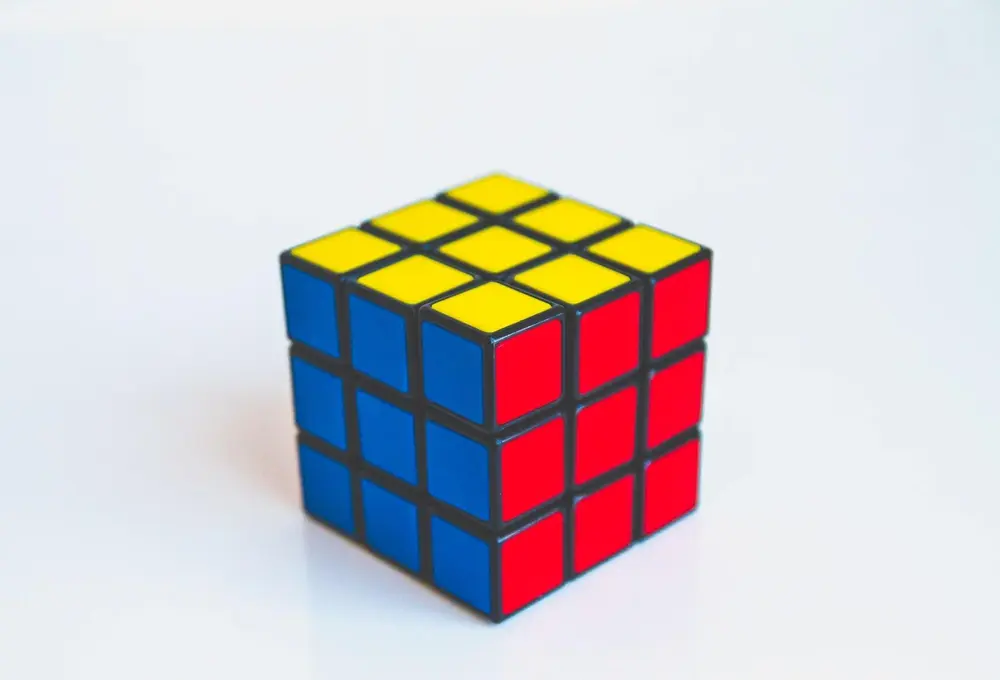# Loci

The locus means the area or line which satisfies a condition. Below are several examples:

The points which are x away from point A.
The locus is a circle around point A with a radius of x.

All the points which are y from rectangle B.
This gives a locus which is y from the straight edges of the rectangle but has a quarter of a circle at the vertices which join the straight lines.

All the points w from the line along cd.
This locus is two lines. One above and one below the line cd. Both are parallel to cd.

The points which are equidistant from the points M and N.
This locus is the perpendicular bisector of the line MN.

Shape and Space

Shape and Space

Shape and Space

Shape and Space

Shape and Space

Shape and Space